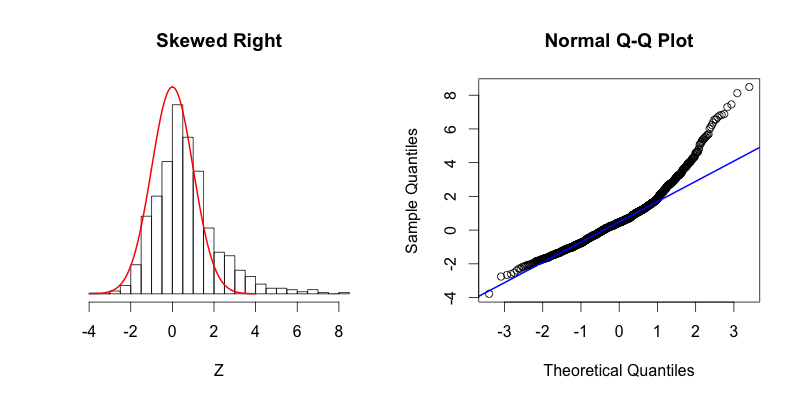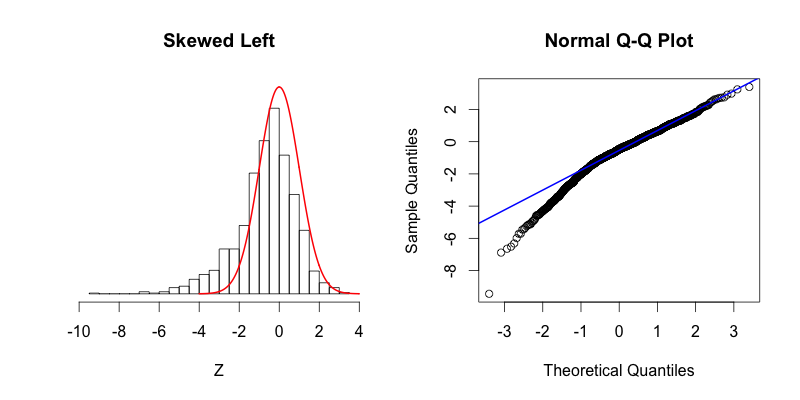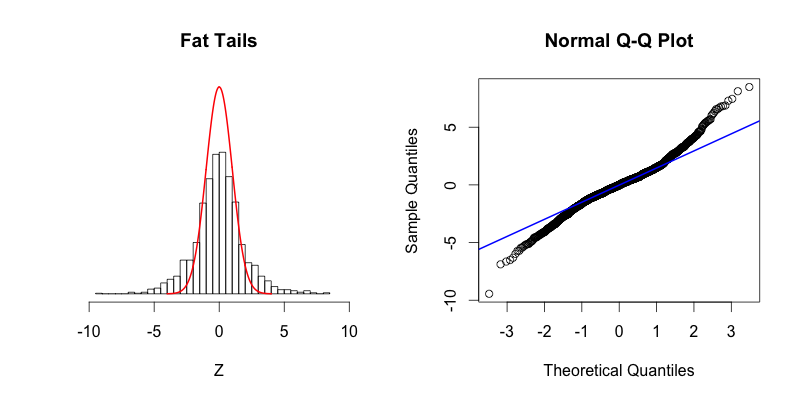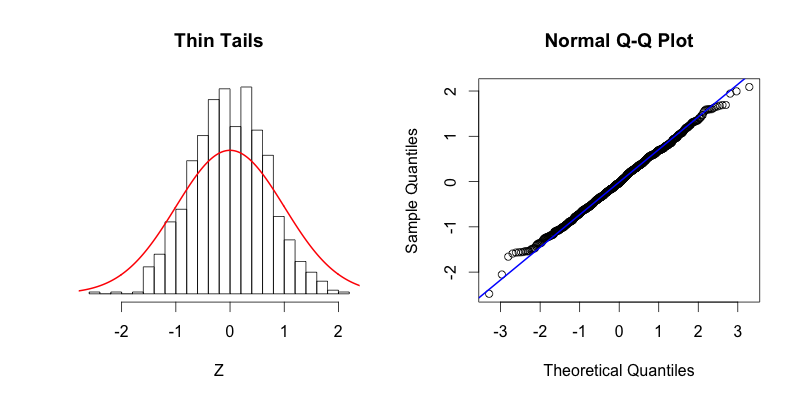# 1 Linear regression model

$Y_i = \beta_0 + \beta_1 X_{i1} + \beta_2 X_{i2} + \ldots + \beta_p X_{ip} + \epsilon_i,\ i = 1,\ldots,n$ where the error $$\epsilon_i$$ is a Gaussian random variable with expectation zero and variance $$\sigma^2$$, $$\epsilon_i \sim^{iid} N(0, \sigma^2)$$.

$E[Y_i] = \beta_0 + \beta_1 X_{i1} + \beta_2 X_{i2} + \ldots + \beta_p X_{ip},\ Var[Y_i]=\sigma^2, \ i = 1,\ldots,n$

In matrix form: $Y \sim N(X\beta, \sigma^2 I)$

In least squares estimation, we find the coefficients $$\beta$$ that minimize the residual sum of squares.

$RSS(\beta) = \sum_{i=1}^n\left(y_i-\sum_{j=0}^p \beta_j x_{ij}\right)^2$

$\hat \beta = (X'X)^{-1}X' y$

$\hat y = X \hat \beta = X (X'X)^{-1}X' y = H y$

## 1.1 Distribution of $$\hat \beta$$

Since $$\hat \beta = (X'X)^{-1}X' Y$$ and $$Y \sim N(X\beta, \sigma^2 I)$$,

$\hat \beta \sim N(\beta, (X'X)^{-1}\sigma^2)$

## 1.2$$\hat \sigma^2$$

$\hat \sigma^2 = \frac{\sum_{i=1}^n (y_i - \hat y_i)^2}{n-p-1}$

## 1.3 Confidence interval for a regression coefficient

$\frac{\hat \beta_j - \beta_j}{se(\hat \beta_j)}= \frac{\hat \beta_j - \beta_j}{\sqrt{\hat \sigma^2 C_{jj}}} \sim t_{n-p-1}$

$$(1-\alpha)100\%$$ confidence interval: $\hat \beta_j \pm t_{n-p-1,\alpha/2} \sqrt{\hat \sigma^2 C_{jj}},$ where $$C_{jj}$$ is the $$j$$th diagonal element of $$(X'X)^{-1}$$.

## 1.4 Hypothesis test for a regression coefficient

To test $$\beta_j = 0$$ we use

$t_j = \frac{\hat \beta_j}{\hat \sigma \sqrt{C_{jj}}},$ where $$C_{jj}$$ is the $$j$$th diagonal element of $$(X'X)^{-1}$$. Under the null hypothesis that $$\beta_j=0$$, $$t_j$$ is distributed as $$t_{n-p-1}$$ and hence a large (absolute) value of $$t_j$$ will lead to rejection of this null hypothesis. If $$\hat \sigma$$ is replaced by a known value $$\sigma$$, then $$t_j$$ would have a standard normal distribution. The difference between the tail quantiles of a t-distribution and a standard normal become negligible as the sample size increases, and so we typically use the normal quantiles.

## 1.5 Hypothesis test for a group of coefficients

To test for the significance of groups of coefficients simultaneously we use the F-test.

$F=\frac{(RSS_0-RSS_1)/(p_1-p_0)}{RSS_1/(n-p_1-1)},$

where $$RSS_1$$ is the residual sum-of-squares for the least squares fit of the bigger model with $$p_1+1$$ parameters, and $$RSS_0$$ the same for the nested smaller model with $$p_0 + 1$$ parameters.

The F statistic measures the change in residual sum-of-squares per additional parameter in the bigger model, and it is normalized by an estimate of $$\sigma^2$$. Under the Gaussian assumptions, and the null hypothesis that the smaller model is correct, the F statistic will have a $$F_{p_1-p_0, n-p_1-1}$$ distribution.

# 2 Assumptions

Linear assumption:

The relationship between the outcomes and the predictors is (approximately) linear.

Error assumptions:

• The errors are independent
• The error term $$\epsilon$$ has constant variance
• The errors are normally distributed

Influential observations:

Occasionally, a few observations may not fit the model well. These have the potential to dramatically alter the results, so we should check for them and investigate their validity.

We should always check fitted models to make sure that these assumptions have not been violated. Diagnostic methods are based primarily on the residuals which are defined as

$e_i = y_i - \hat y_i,\ i=1, \ldots,n$

Residual analysis is usually done graphically. We may look at quantile plots to assess normality, and scatterplots to assess assumptions such as constant variance and linearity, and to identify potential outliers.

Standardized residual

Standardized residual is an ordinary residual divided by an estimate of its standard deviation.

$e_i = y_i - \hat{y_i}$

$r_i = \frac{e_i}{se(e_i)} = \frac{e_i}{\hat \sigma \sqrt{1-h_{ii}}} = \frac{e_i}{ \sqrt{MSE (1-h_{ii})}}$ Standardized residuals quantify how large the residuals are in standard deviation units, and therefore can be easily used to identify outliers.

An observation with a standardized residual that is larger than 2 or 3 (in absolute value) is deemed by some to be an outlier.

When trying to identify outliers, one problem that can arise is when there is a potential outlier that influences the regression model to such an extent that the estimated regression function is “pulled” towards the potential outlier, so that it is not flagged as an outlier using the standardized residual criterion.

## 2.1 Normality assumption. Review Q-Q plot

The residuals from the model provide estimates of the random errors. If the normality assumption is met, then the residuals all-together should approximately follow a normal distribution.

We check normality of errors with a normal quantile-quantile (Q-Q) plot of the residuals.

The normal quantile-quantile (Q-Q) plot is a visual assessment of how well residuals match what we would expect from a normal distribution. Outliers, skew, heavy and light-tailed aspects of distributions (all violations of normality) will show up in this plot.

Example: Fit simple linear regression model to investigate the relationship between physical activity (PA) and body mass index (BMI)

d <- read.table("data/pabmi.txt", header = TRUE)
head(d)
summary(d)
##     SUBJECT             PA              BMI
##  Min.   :  1.00   Min.   : 3.186   Min.   :14.20
##  1st Qu.: 25.75   1st Qu.: 6.803   1st Qu.:21.10
##  Median : 50.50   Median : 8.409   Median :24.45
##  Mean   : 50.50   Mean   : 8.614   Mean   :23.94
##  3rd Qu.: 75.25   3rd Qu.:10.274   3rd Qu.:26.75
##  Max.   :100.00   Max.   :14.209   Max.   :35.10
plot(d$PA, d$BMI)res <- lm(BMI ~ PA, data = d)
summary(res)
##
## Call:
## lm(formula = BMI ~ PA, data = d)
##
## Residuals:
##     Min      1Q  Median      3Q     Max
## -7.3819 -2.5636  0.2062  1.9820  8.5078
##
## Coefficients:
##             Estimate Std. Error t value Pr(>|t|)
## (Intercept)  29.5782     1.4120  20.948  < 2e-16 ***
## PA           -0.6547     0.1583  -4.135  7.5e-05 ***
## ---
## Signif. codes:  0 '***' 0.001 '**' 0.01 '*' 0.05 '.' 0.1 ' ' 1
##
## Residual standard error: 3.655 on 98 degrees of freedom
## Multiple R-squared:  0.1485, Adjusted R-squared:  0.1399
## F-statistic:  17.1 on 1 and 98 DF,  p-value: 7.503e-05

We can obtain the Q-Q plot for the residuals of the regression model as follows:

plot(res, 2)A Q-Q plot compares the data with what to expect to get if the theoretical distribution from which the data come is normal.

The Q-Q plot displays the value of observed quantiles in the standardized residual distribution on the y-axis versus the quantiles of the theoretical normal distribution on the x-axis.

• If data are normally distributed, we should see the plotted points lie close to the straight line.
• If we see a concave normal probability plot, log transforming the response variable may remove the problem.

### 2.1.1 Q-Q plot

The Q-Q plot is a graphical technique for determining if two data sets come from populations with a common distribution. A Q-Q plot is a plot of the quantiles of the first data set against the quantiles of the second data set.

A quantile is the value at which a given percent of points is below that value. That is, the 0.3 quantile is the value at which 30% percent of the data fall below and 70% fall above that value.

The Q-Q-plot is formed by:
Vertical axis: Estimated quantiles from data set 1
Horizontal axis: Estimated quantiles from data set 2
Both axes are in units of their respective data sets. That is, the actual quantile level is not plotted. For a given point on the Q-Q plot, we know that the quantile level is the same for both points, but not what that quantile level actually is.

### 2.1.2 Normal Q-Q plot

A Normal Q-Q plot shows the “match” of an observed distribution with the theoretical normal distribution.

The Q-Q plots display the value of observed quantiles in the standardized residual distribution on the y-axis versus the quantiles of the theoretical normal distribution on the x-axis.

If the observed distribution of the residuals matches the shape of the normal distribution, then the plotted points should follow a 1-1 relationship.

If the points follow the displayed straight line that suggests that the residuals have a similar shape to a normal distribution. Some variation is expected around the line and some patterns of deviation are worse than others for our models.

### 2.1.3 Examples

Normal distribution is also called Gaussian distribution

• NormalThe blue line shows where the points would fall if the dataset were normally distributed.

The points in the Q-Q plot form a relatively straight line since the quantiles of the dataset nearly match what the quantiles of the dataset would theoretically be if the dataset was normally distributed

• Skewed rightSkewed right, most of the data is distributed on the left side with a long tail of data extending out to the right.

The last two theoretical quantiles for this dataset should be around 3, when in fact those quantiles are greater than 8.

The point’s trend upward shows that the actual quantiles are much greater than the theoretical quantiles, meaning that there is a greater concentration of data beyond the right side of a Gaussian distribution.

• Skewed leftSkewed left, most of the data is distributed on the right side with a long tail of data extending out to the left.

There is more data to the left of the Gaussian distribution. The points appear below the blue line because those quantiles occur at much lower values (between -9 and -4) compared to where those quantiles would be in a Gaussian distribution (between -4 and -2).

• Fat tailsFat tails, compared to the normal distribution there is more data located at the extremes of the distribution and less data in the center of the distribution.

In terms of quantiles this means that the first quantile is much less than the first theoretical quantile and the last quantile is greater than the last theoretical quantile.

• Thin tailsThin tails, there is more data concentrated in the center of the distribution and less data in the tails. These thin tails correspond to the first quantiles occurring at larger than expected values and the last quantiles occurring at less than expected values.